SBOS703F April   2014  – October 2016

PRODUCTION DATA.

1. Features
2. Applications
3. Description
4. Revision History
5. Pin Configuration and Functions
6. Specifications
7. Detailed Description
1. 7.1 Overview
2. 7.2 Functional Block Diagram
3. 7.3 Feature Description
4. 7.4 Device Functional Modes
8. Application and Implementation
1. 8.1 Application Information
2. 8.2 Typical Application
9. Power Supply Recommendations
10. 10Layout
11. 11Device and Documentation Support
12. 12Mechanical, Packaging, and Orderable Information
13. 11Mechanical, Packaging, and Orderable Information

• PW|14
• D|14
• PW|14
• D|14

## 8 Application and Implementation

NOTE

Information in the following applications sections is not part of the TI component specification, and TI does not warrant its accuracy or completeness. TI’s customers are responsible for determining suitability of components for their purposes. Customers should validate and test their design implementation to confirm system functionality.

### 8.1 Application Information

#### 8.1.1 General Configurations

When receiving low-level signals, the device often requires limiting the bandwidth of the incoming signals into the system. The simplest way to establish this limited bandwidth is to place an RC filter at the noninverting pin of the amplifier, as Figure 39 shows.Figure 39. Single-Pole Low-Pass Filter

If even more attenuation is needed, the device requires a multiple-pole filter. The Sallen-Key filter can be used for this task, as Figure 40 shows. For best results, the amplifier must have a bandwidth that is 8 to 10 times the filter frequency bandwidth. Failure to follow this guideline can result in phase shift of the amplifier.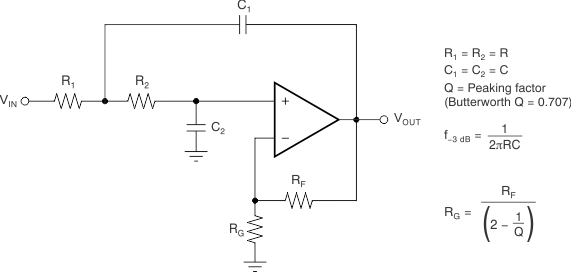Figure 40. Two-Pole, Low-Pass, Sallen-Key Filter

### 8.2 Typical Application

Some applications require differential signals. Figure 41 shows a simple circuit to convert a single-ended input of 0.1 V to 2.4 V into a differential output of ±2.3 V on a single 2.7-V supply. The output range is intentionally limited to maximize linearity. The circuit is composed of two amplifiers. One amplifier functions as a buffer and creates a voltage, VOUT+. The second amplifier inverts the input and adds a reference voltage to generate VOUT–. VOUT+ and VOUT– range from 0.1 V to 2.4 V. The difference, VDIFF, is the difference between VOUT+ and VOUT– which makes the differential output voltage range 2.3 V.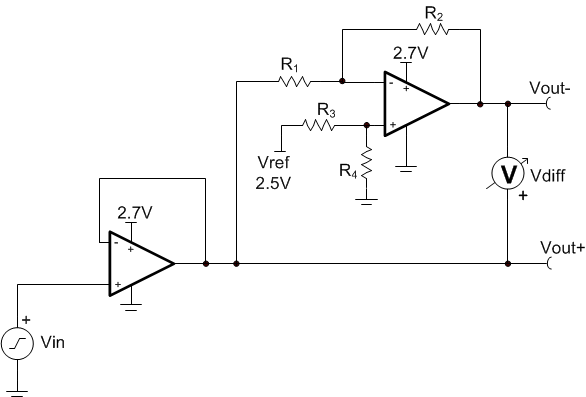Figure 41. Schematic for a Single-Ended Input to Differential Output Conversion

#### 8.2.1 Design Requirements

Table 1 lists the design requirements:

### Table 1. Design Parameters

DESIGN PARAMETER VALUE
Supply voltage 2.7 V
Reference voltage 2.5 V
Input voltage 0.1 V to 2.4 V
Output differential voltage ±2.3 V
Output common-mode voltage 1.25 V
Small-signal bandwidth 5 MHz

#### 8.2.2 Detailed Design Procedure

The circuit in Figure 41 takes a single-ended input signal, VIN, and generates two output signals, VOUT+ and VOUT– using two amplifiers and a reference voltage, VREF. VOUT+ is the output of the first amplifier and is a buffered version of the input signal, VIN (as shown in Equation 1). VOUT– is the output of the second amplifier which uses VREF to add an offset voltage to VIN and feedback to add inverting gain. The transfer function for VOUT– is given in Equation 2.

Equation 1.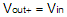Equation 2.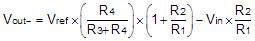The differential output signal, VDIFF, is the difference between the two single-ended output signals, VOUT+ and VOUT–. Equation 3 shows the transfer function for VDIFF. Using conditions in Equation 4 and Equation 5 and applying the conditions that R1 = R2 and R3 = R4, the transfer function is simplified into Equation 6. Using this configuration, the maximum input signal is equal to the reference voltage, and the maximum output of each amplifier is equal to VREF. The differential output range is 2 × VREF. Furthermore, the common-mode voltage is one half of VREF, as shown in Equation 7.

Equation 3.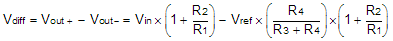Equation 4.Equation 5.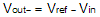Equation 6.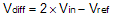Equation 7.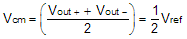#### 8.2.2.1 Amplifier Selection

Linearity over the input range is key for good dc accuracy. The common-mode input range and output swing limitations determine the linearity. In general, an amplifier with rail-to-rail input and output swing is required. Bandwidth is a key concern for this design, so the OPAx316 is selected because the bandwidth is greater than the target of 5 MHz. The bandwidth and power ratio makes this device power efficient and the low offset and drift ensure good accuracy for moderate precision applications.

#### 8.2.2.2 Passive Component Selection

Because the transfer function of VOUT– is heavily reliant on resistors (R1, R2, R3, and R4), use resistors with low tolerances to maximize performance and minimize error. This design uses resistors with resistance values of 49.9 kΩ and tolerances of 0.1%. However, if the noise of the system is a key parameter, smaller resistance values (6 kΩ or lower) can be selected to keep the overall system noise low. This ensures that the noise from the resistors is lower than the amplifier noise.

#### 8.2.3 Application Curves

The measured transfer functions in Figure 42, Figure 43, and Figure 44 are generated by sweeping the input voltage from 0.1 V to 2.4 V. The full input range is actually 0 V to 2.5 V, but is restricted by 0.1 V to maintain optimal linearity. For more details on this design and other alternative devices that can be used in place of the OPAx316, see (Single-Ended Input to Differential Output Conversion Circuit Reference Design (TIPD131).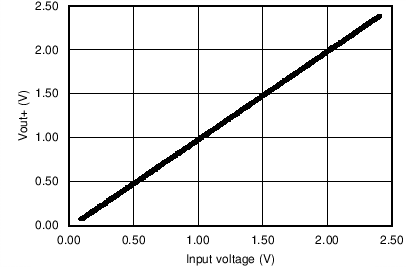Figure 42. VOUT+ vs Input Voltage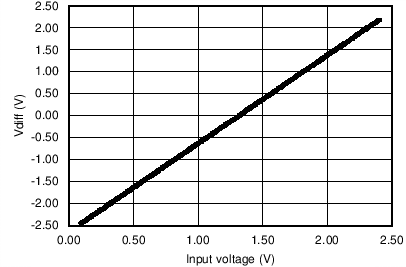Figure 44. VDIFF vs Input Voltage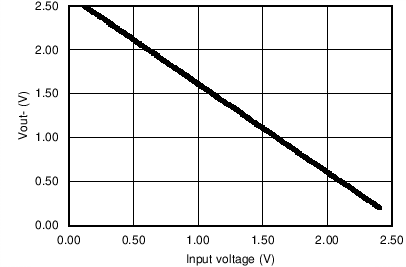Figure 43. VOUT– vs Input Voltage